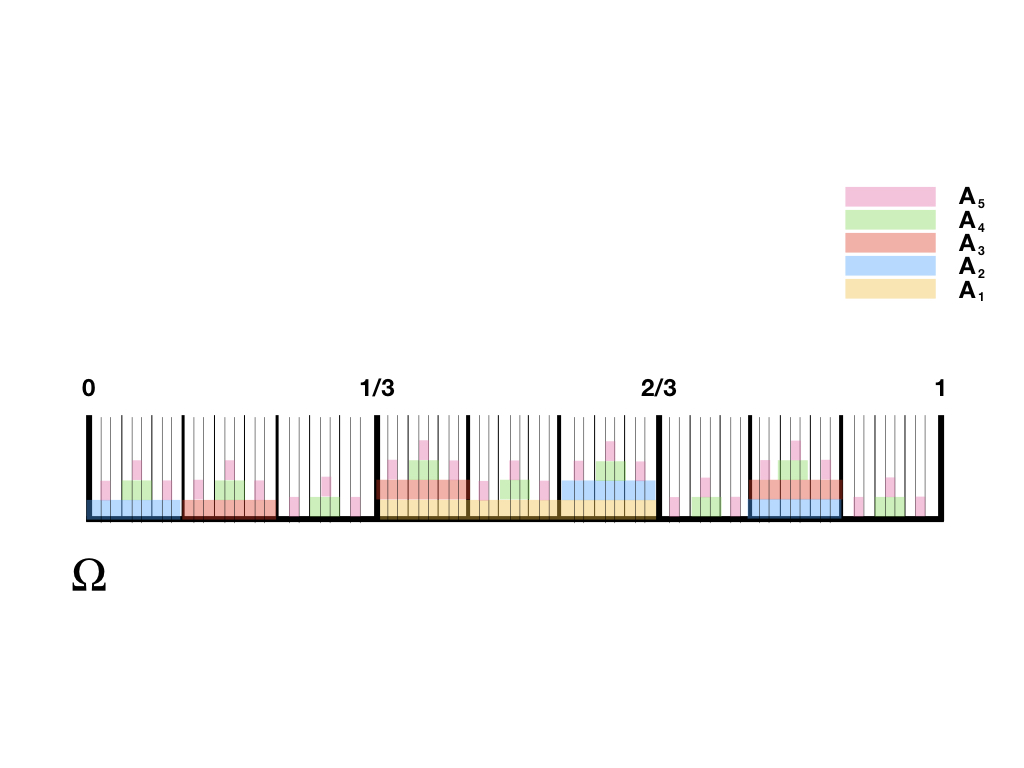# Pairwise independence does not imply countable mutual independenceDefine a sequence of random variables indexed by $$n$$ as follows:

1. Divide the unit interval into sub-intervals of size $$1/3^n$$

2. Let the random variable be equal to $$1$$ if $$\omega$$ falls within the “middle” third of each subinterval, and let it be equal to $$0$$ otherwise

More formally, let

$X_n := \mathbf{1}_{B_n}$

where $$B_n$$ is the event defined as

$B_n := \Bigg\{\omega : \omega \in \bigcup_{j = 1}^{3^{n-1}} \bigg( \frac{3(j-1)+1}{3^{n}}, \frac{3(j-1)+2}{3^{n}} \bigg] \Bigg\}$

Following this procedure, one gets an iid sequence of random variables

$\{X_n\} \overset{\text{iid}}{\sim} \text{Bernoulli}\Big(\frac{1}{3}\Big)$

Independence follows from the fact that

$\mathsf{P}\big[\cap_{i \in \mathcal{I}} B_i \big] = \prod_{i \in \mathcal{I}}\mathsf{P}\big[B_i \big]$

for any index set $$\mathcal{I}$$. Identical distribution follows from the fact that

$\mathsf{P}[X_i = 1] = 1/3$

for each $$i$$.

Mutual independence, however, is a delicate thing. A small deviation from the setup above can result in a sequence of random variables that are pairwise independent, but not mutually independent.

We can change the position of two of the events from the previous construction to create a sequence of pairwise independent, but not mutually independent, Bernoulli random variables.

To see this, let

$Y_n := \mathbf{1}_{A_n}$

where $$A_n$$ is defined as follows:

For $$n=1$$, divide the unit interval into $$3$$ intervals of equal size and set

\begin{aligned} A_1 := (1/3,2/3] \end{aligned}

For $$n = 2$$ and $$n=3$$, define

\begin{aligned} A_2 &:= (0,1/9] \cup (5/9,2/3] \cup (7/9,8/9] \\ A_3 &:= (1/9,2/9] \cup (1/3,4/9] \cup (7/9,8/9] \end{aligned}

For each $$n > 3$$, divide the interval $$(0,1]$$ into $$\frac{1}{3^{n-1}}$$ subintervals of equal size, and define $$A_n$$ to be all $$\omega$$ that fall within the middle third of each of these segments, as before.

The figure at the top of the page illustrates events $$A_1, \dots, A_5$$ under this scheme. Intuitively, one can see that the events cannot be mutually independent because there is no place on the unit interval where all five colours overlap. Namely,

\begin{aligned} \mathsf{P}\big[\cap_{i=1}^n A_i \big] &= \mathsf{P}\big[\varnothing \big] = 0 \end{aligned}

which is not equal to

$\prod_{i=1}^n \mathsf{P}\big[A_i \big] = 1 / 3^n$

However, any set of more than three events $$\{ A_i \}$$ that does not contain all three of $$A_1, A_2, A_3$$ will be mutually independent. In this particular construction, it is only the three-way interaction of $$A_1, A_2, A_3$$ that destroys the mutual independence of the full sequence.